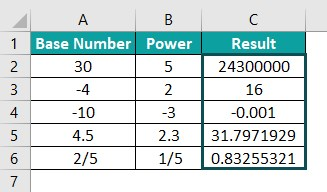# POWER Function In ExcelArticle byExcelMojo Team## What Is POWER Function In Excel?

The POWER function in Excel is an inbuilt Math & Trig function that returns the given number raised to the specified power. It is a function used as an alternative to the exponent operator.

Users can use POWER() in financial and mathematical calculations, such as determining compound interest and evaluating algebraic expressions in Excel.

For example, the table below contains a list of numbers in column A and the powers in column B to which we require to raise them.

Suppose we have to display the results in column D cells D2:D7. Then using POWER function in Excel in the target cells, we can achieve the desired output.

The above example shows different scenarios where we can use the function. For instance, the number and power value can be positive or negative integer, fractional value, or decimal numbers.

###### Key Takeaways
• The POWER function in Excel determines a number, the given base number raised to the specified power. Users can apply the POWER() when performing financial and mathematical evaluations involving exponential terms.
• The POWER syntax is =POWER(number, power) where,
• number is the real number we require to raise to a given power value.
• power is the exponent to which we require to raise the specified numeric value.
• Both the arguments in the POWER() are mandatory. Also, the arguments can be positive or negative integers, fractional values, or decimal numbers.
• Remember, we can achieve fruitful results using the POWER() with other Excel functions such as IF.

### POWER() Excel Formula

The POWER formula in Excel is:

where,

• number: A real number we require to raise to a given power value.
• power: The exponent to which we require to raise the specified numeric value.

The above two arguments in the POWER formula are mandatory. They can be numeric values or excel cell references to the numeric values.

Please Note: The POWER formula in Excel works only for numeric values. If we provide non-numeric values as arguments to the function, it returns #VALUE! error.

### How To Use POWER Excel Function?

The steps to use the POWER function in Excel are:

1. First, ensure the number and power values in the source data are numeric values.

2. Then, select the target cell and enter the POWER function.

3. Finally, press Enter to view the output, which will be the given number raised to the specified power.

Below is an example to understand the POWER function and the above steps.

Consider the below table. It contains real numbers and the respective powers to which we require to raise them.

Please Note: When entering fractional values in the source data, ensure the data format in the Home tab is Fraction. Otherwise, Excel will assume it as a date value, and the POWER() will return the #NUM! error.

We can populate the required data in the target cell range C2:C11 using POWER function in Excel.

Step 1: To begin with, select the target cell C2, enter the POWER() provided in the Formula Bar in the image below, and then, press Enter.

Alternatively, we can select the target cell C2 and then, click Formulas à Math & Trig à POWER to open the Function Arguments window.

Next, enter the two argument values in the Function Arguments window.

While the Function Arguments window shows the POWER function in Excel, once we click OK, we can view the result it displays in the target cell C2, 1.709975947.

Step 2: Next, drag the fill handle downwards to copy the formula in cell range C3:C11.

We can also enter the POWER() in each cell individually. Now, let us consider the target cell C11. Meanwhile, we can also type the formula in the following ways:

=POWER(A11,3)

or

=POWER(50,B11)

or

=POWER(50,3)

Meanwhile, the POWER() return value will be the same in each case, 125000.

The POWER() follows the mathematical way of calculating an exponent value. For example, in row 7, the number argument is 30, and the power argument is -1. Thus, the POWER() calculates (1/30)^1, thus returning the value 0.033333333.

### Examples

Here are some scenarios where we can apply the POWER function in Excel.

#### Example #1

The mathematical formula for compound interest is:

where,

• A: Final amount
• P: Initial principal
• r: Interest rate
• n: Number of times interest gets compounded per unit period, t.
• t: Number of periods passed.

Suppose we have to evaluate the above compound interest formula for the below data in Excel and display the final amount in the target cell D9.

Then, we can apply the POWER function in Excel formula in cell D9 to achieve the required compound interest amount.

Please Note: The cell D4 containing the principal value has the data format set as Currency. And the rate value in cell D5 is a decimal value to make the calculations straightforward.

1: First, select the target cell D9, enter the below formula containing the POWER(), and then, press Enter.

=D4*POWER((1+(D5/D7)),(D7*D6))

2: Next, select cell D9 and set the data format in the Home tab as Currency.

Once we click the Currency option, we can clearly see the final amount will be in the proper format.

In the above formula, the POWER function in Excel executes first, and its return value gets multiplied by the principal amount, \$5,000.00. Here is how the calculation happens.

=5000*POWER((1+(0.15/12)),(D7*D6))

=5000*POWER((1+0.0125),( D7*D6))

=5000*POWER(1.0125,( D7*D6))

=5000*POWER(1.0125,(12*5))

=5000*POWER(1.0125,60)

=5000*2.10718134695124

=10535.90673

#### Example #2

In this example, we shall see how we can use the function to solve algebraic problems.

In the above expression, x is an unknown value, and a, b, and c are the coefficients. And solving the above equation involves finding its two roots.

Suppose we have quadratic equations to solve in Excel. The equations are in column A, and now, we must display their roots in the target columns B and C.

Then we can use the POWER function in Excel to update the roots for each equation in the target cells.

Now, consider the first equation: x2 + 4x – 5 = 0

For the above equation, a = 1, b = 4, c = -5. Thus, the roots are:

Here is how we can apply the formula containing the POWER function in Excel to execute the above mathematical calculations in the target cell range B2:C6.

1: To begin with, select the target cell B2, enter the below formula, and then, press Enter.

=((-4+POWER(POWER(4,2)-(4*1*(-5)),1/2)))/(2*1)

In the above formula, the inner POWER() returns the square of 4, 16. Then the number argument of the outer POWER() becomes 36. So the outer POWER() returns the square root of 36, 6. And finally, solving the remaining equation results in the value 1.

2: Next select the target cell C2, enter the below formula, and press Enter.

=((-4-POWER(POWER(4,2)-(4*1*(-5)),1/2)))/(2*1)

3: Similarly, substitute the coefficient values a, b, and c in the roots 1 and 2 formulas containing the POWER() for each quadratic equation in cell range A3:A6.

And the final output will be:

#### Example #3

Now, we can use the POWER function in Excel with other inbuilt functions such as IF excel function to achieve the desired results.

Assume the table contains a list of base numbers.

And the requirement is to determine the square of a base number if it is less than or equal to 5. Otherwise, we need to find the cube of the base number. So, the steps are:

1: First, select the target cell B2, enter the below formula containing the POWER function in Excel within an IF condition, and then, press Enter.

=IF(A2<=5,POWER(A2,2),POWER(A2,3))

As the base number 1 is less than 5, the IF condition holds in the target cell B2. And so, the POWER() determines the square of 1, 1, which the formula returns as the output.

2: Next, drag the fill handle downwards to copy the formula in cell range B3:B11.

Thus, the output shows the square of numbers from 1 to 5 and the cube of numbers from 6 to 10.

### Important Things To Note

• We can use the POWER function in Excelas an alternative to the exponent operator (^).
• First, ensure the POWER() arguments are numeric values or cell references to numeric values. Otherwise, the function output will be the #VALUE! error.
• If the POWER() argument value is a fraction, ensure we set the specific cell data format in the Home tab as Fraction. Otherwise, the function will throw the #NUM! error.
• The POWER() makes determining values such as compound interests and solving mathematical problems involving algebraic expressions straightforward.

1. Where is the POWER function in Excel?

The POWER function is in the Formulas tab. So, click FormulasMath & Trig POWER to access it.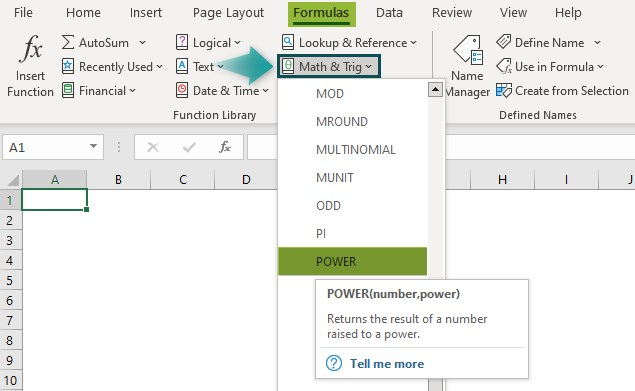2. How to graph a POWER function in Excel?

We can graph a POWER function in Excel as explained below. Let us see the steps with an example.
Consider the table below. It shows a list of base numbers, and the power value is 3.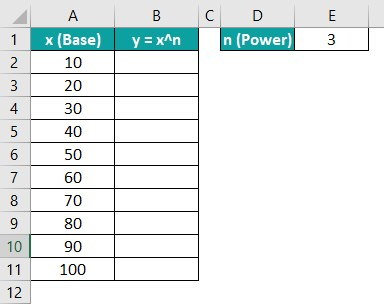Suppose we must find the cube of the listed base numbers, x, display the cube values, y, in the target cell range B2:B11, and plot a graph for the resulting cubes. Then, we can achieve the desired outcome by applying the POWER function in the target cells and creating the required graph by following the below steps.

Step 1: First, select the target cell B2, enter the POWER() provided in the Formula Bar in the image below, and then, press Enter.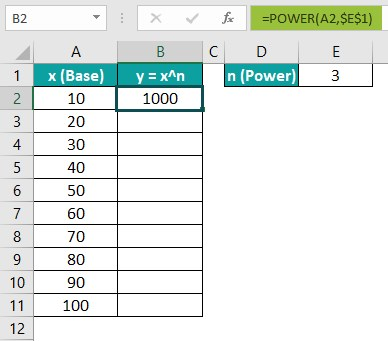Step 2: Next, drag the fill handle downwards to copy the formula in cell range B3:B11.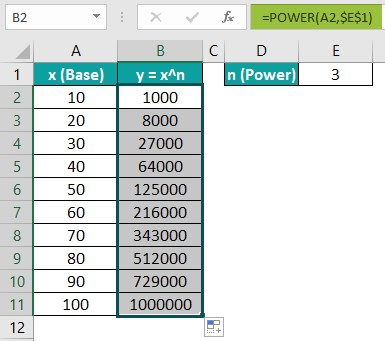Step 3: Then, select the cell range A1:B11, and click InsertScatterScatter with Smooth Lines and Markers.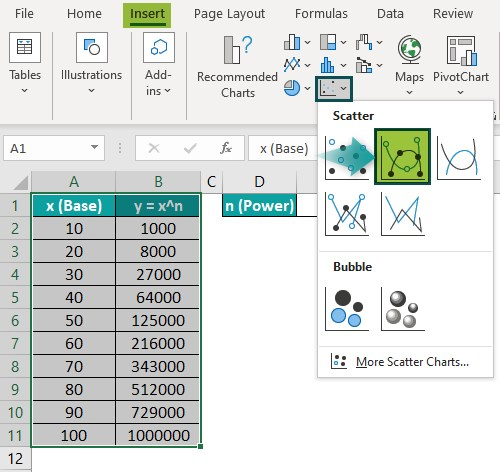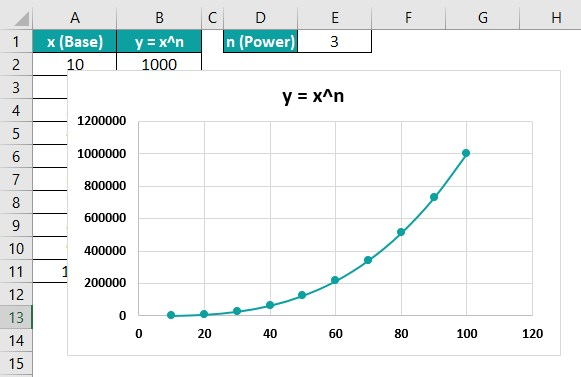Step 4: Now, click on the resulting exponential chart area to access the Chart Elements (+) option.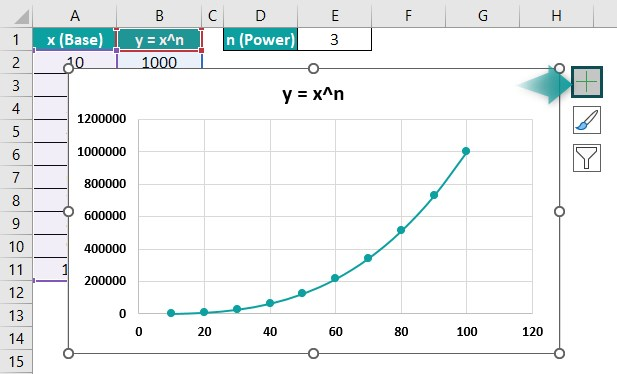Step 5: Similarly, click the Chart Elements (+) option to update the Axis Titles and Data Labels.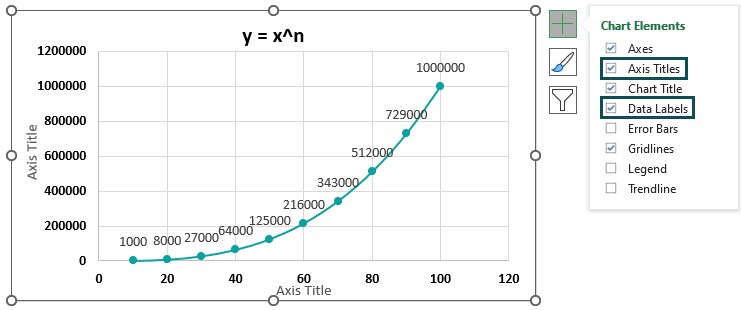And once we update the chart title and the axes titles, the final exponential chart for the POWER() will be: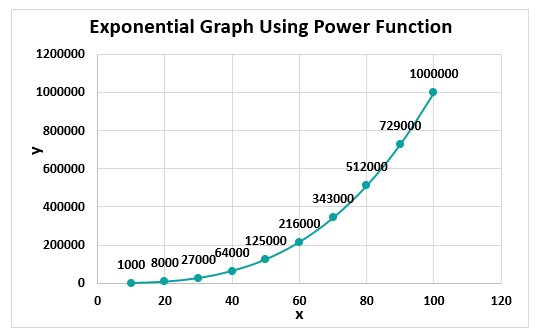3. How to use the POWER() in Excel VBA?

We can use the POWER() in Excel VBA by following the below steps. Let us understand them with an example.

Suppose the following table contains a list of base numbers and the powers to which we require to raise them. And also, we have to display the output in cell range C2:C6.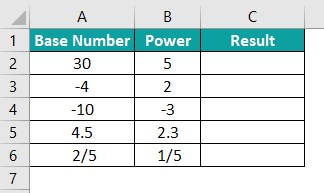We can use the POWER function in Excel VBA to populate the required results in the target cells.

Step 1: First, press the keyboard shortcut Alt + F11 with the worksheet containing the above table open, to open the VBA Editor window.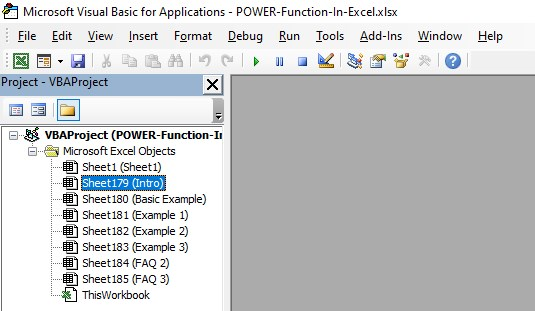Step 2: Next, select the required VBA Project and click InsertModule to open the Module1 window.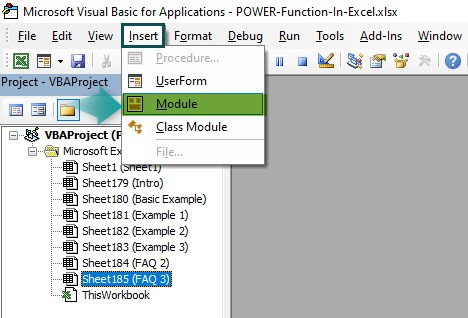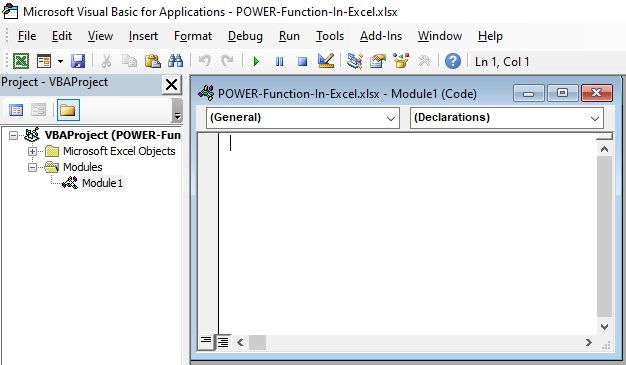Step 3: Now, enter the code to apply the POWER() in Excel using VBA in the Module1 window.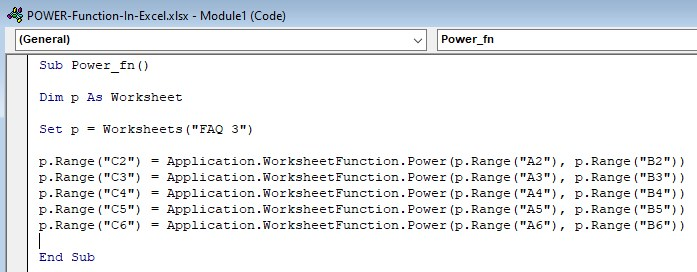Step 4: Next, click on the Run Sub/UserForm icon in the menu to run the commands.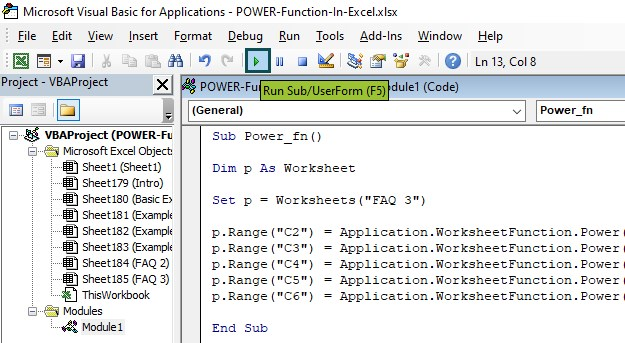And once we execute the POWER function in Excel VBA commands, we can clearly see the output as shown in the below image.